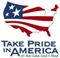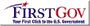Scientific Investigations Report 2007–5008

U.S. GEOLOGICAL SURVEY
Scientific Investigations Report 2007–5008

## Conversion Factors and Datums

### SI to Inch-Pound

Multiply By To obtain
centimeter (cm) 0.394 inch (in.)
centimeter per second (cm/s) 0.394 inch per second (in/s)
centimeter per square second (cm/s2) 0.394 inch per square second (in/s2)
cubic meter (m3) 264.2 gallon (gal)
cubic meter (m3) 0.0008107 acre-foot (acre-ft)
gram per cubic centimeter (g/cm3) 62.4220 pound per cubic foot (lb/ft3)
kilometer (km) 0.6214 mile (mi)
kilogram (kg) 2.205 pound avoirdupois (lb)
kilogram per square meter (kg/m2) 0.2048 pound per square foot (lb/ft2)
meter (m) 3.281 foot (ft)
meter per day (m/d) 3.281 foot per day (ft/d)
metric ton 1.102 ton
square meter per gram (m2/g) 4882 square foot per pount (ft2/lb)
square meter per second (m2/s) 10.76 square foot per second (ft2/s)
square kilometer (km2) 247.1 acre
square centimeter (cm2) 0.001076 square foot (ft2)
square meter (m2) 10.76 square foot (ft2)
square centimeter (cm2) 0.1550
square kilometer (km2) 0.3861 square mile (mi2)
Watt per square meter per second [(W/m2)/s] 0.317 BTU per square foot per second [(BTU/ft2)/s]

Temperature in degrees Celsius (°C) may be converted to degrees Fahrenheit (°F) as follows:

°F=(1.8×°C)+32

Specific conductance is given in microsiemens per centimeter at 25 degrees Celsius (µS/cm at 25 °C).

Concentrations of chemical constituents in water generally are given in milligrams per liter (mg/L), which is approximately equal to parts per million.

Turbidity is given in Formazin Nephelometric Units, or FNUs.

Datum

Horizontal coordinate information is referenced to North American Datum of 1927 (NAD27).

Vertical coordinate information is referenced to the National Geodetic Vertical Datum of 1929 (NGVD29).U.S. Department of the Interior | U.S. Geological Survey